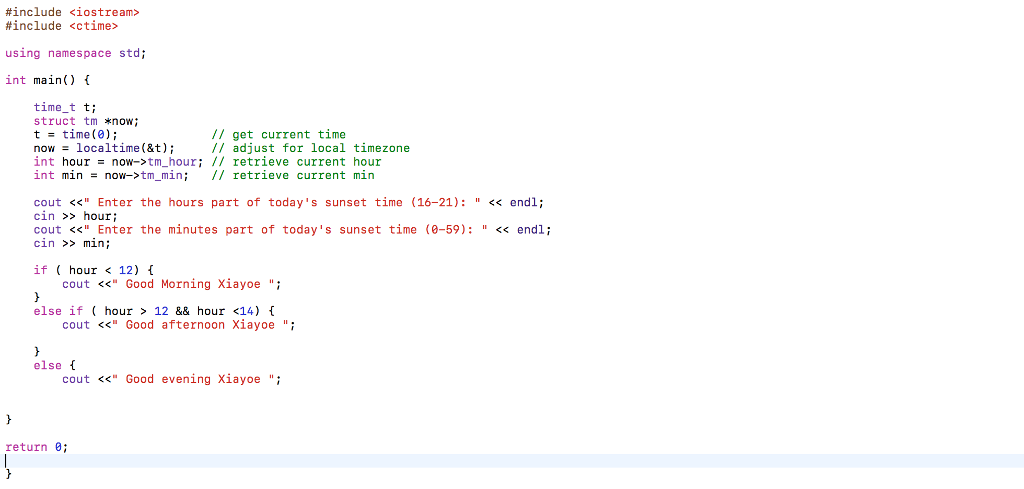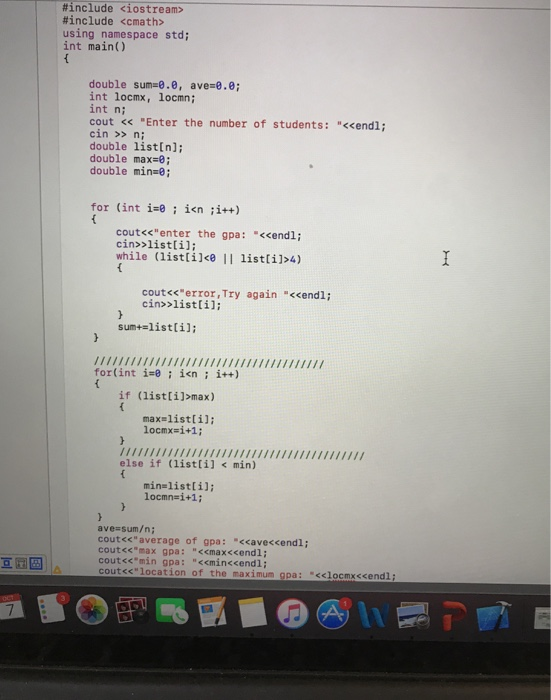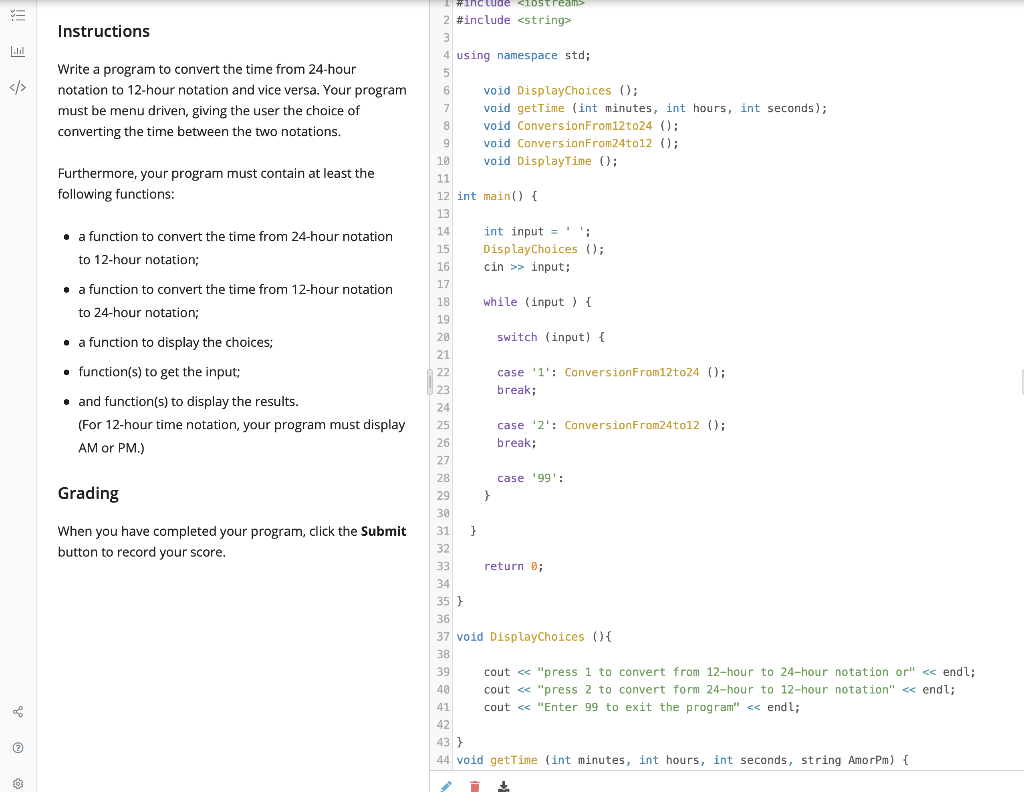# I have a program that needs comments added to it: #include <iostream> #include <stdio.h> #include <conio.h>...

#include <iostream>
#include <stdio.h>
#include <conio.h>
#include <windows.h>
using namespace std;

int main()
{
int m, s,h;
cout << "A COUNTDOWN TIMER " << endl;
cout << "enter time in hours here" << endl;
cin >> h;
cout << "enter time in minutes here " << endl;
cin >> m;
cout << "enter time in seconds here" << endl;
cin >> s;
cout << "Press any key to start" << endl;
cout << " A COUNTDOWN TIMER" << endl;
cout << "time remaining" << endl;
cout << "hours : " << h << "mins : " << m << " secs : " << s << endl;
for (int hour = h; hour >= 0; hour--)
{
for (int min = m; min >= 0 ; min--)
{
if ( min == 0 && h > 0)
m = 59;
for (int sec = s; sec >= 0; sec--)
{
if ( sec == 0 )
s = 59;
Sleep(1000);
system("clear");
cout << hour << " :hours " << min << " :mins " << sec << " :secs" << endl;
}
}
}

Sleep(1000);
cout << "THE END" << endl;

return 0;
}

This Homework Help Question: "I have a program that needs comments added to it: #include <iostream> #include <stdio.h> #include <conio.h>..." No answers yet.

We need 10 more requests to produce the answer to this homework help question. Share with your friends to get the answer faster!

0 /10 have requested the answer to this homework help question.

Once 10 people have made a request, the answer to this question will be available in 1-2 days.
All students who have requested the answer will be notified once they are available.

#### Earn Coin

Coins can be redeemed for fabulous gifts.

Similar Homework Help Questions
• ### How can I modify the program below with this prompt asking "How many minutes from now...How can I modify the program below with this prompt asking "How many minutes from now do you expect to be home?", and output a sentence saying "You will get home at HH:MM". ?? It's very URGENT!! Thank you #include <iostream> #include <ctime> using namespace std; int main) time t t struct tm *now t-time (e) now-localtime(&t); int hour = now->tm.hour; // retrieve current hour int min = now->tm..min; // retrieve current min // get current time // adjust for...

• ### fully comments for my program, thank you will thumb up #include <iostream> #include <fstream> #include <string>...

fully comments for my program, thank you will thumb up #include <iostream> #include <fstream> #include <string> #include <iomanip> using namespace std; struct book { int ISBN; string Author; string Title; string publisher; int Quantity; double price; }; void choice1(book books[], int& size, int MAX_SIZE) { ifstream inFile; inFile.open("inventory.txt"); if (inFile.fail()) cout <<"file could not open"<<endl; string str;    while(inFile && size < MAX_SIZE) { getline(inFile, str); books[size].ISBN = atoi(str.c_str()); getline(inFile, books[size].Title);    getline(inFile, books[size].Author); getline(inFile, books[size].publisher);          getline(inFile,...

• ### Flow chart of this program #include <iostream> #include <cmath> using namespace std; int mainO double sum=0.0,...Flow chart of this program #include <iostream> #include <cmath> using namespace std; int mainO double sum=0.0, ave-ee, int locmx, locmn int n; cout <<"Enter the number of students: "<<endl; cin >> ni double listln]; double max-0; double min-e; for (int i-e ; i<n ;i++) cout<s enter the gpa: "<cendli cin>>listli]; while (listlile i listli1>4) cout<s error,Try again "<cendl; cin>listlil: sun+=list[i]; N1 if (listli]>max) for(int isin itt) max=list [i]; 10cmx=1+1 ; else if (list [i] min) min=list [i]; locmn-i+1; ave sum/n;...

• ### #include <iostream>#include <string>using namespace std;// Implement printArray here// Implement deleteSmallest here//...

#include#includeusing namespace std;// Implement printArray here// Implement deleteSmallest here// DO NOT CHANGE MAIN FUNCTION BELOWint main() {int myarray;cout << "Enter number of integers : ";int n;cin >> n;cout << "Enter " << n << " integers" << endl;for (int i = 0; i < n; i++)cin >> myarray[i];cout << "Contents of array : ";printArray(myarray, n);deleteSmallest(myarray, n);cout << "Contents of array after deleteSmallest: ";printArray(myarray, n-1);}

• ### graph binary search for size and time c++ //System Libraries #include <iostream> #include <string> #include <cstdlib> #include <ctime> #include <iomanip> #include <alg...

graph binary search for size and time c++ //System Libraries #include <iostream> #include <string> #include <cstdlib> #include <ctime> #include <iomanip> #include <algorithm> using namespace std; //User Libraries //Global Constants, no Global Variables are allowed //Math/Physics/Conversions/Higher Dimensions - i.e. PI, e, etc... //Function Prototypes //Execution Begins Here! int main(int argc, char** argv) { int n, i, arr, search, first, last, middle,count=0,count_in,tot; clock_t start, end; float duration; cout<<"Enter total number of elements :"; cin>>n; cout<<"Enter numbers"; for (i=0; i<n;i++) cin>>arr[i]; cout<<"Enter a...

• ### My if/else statement wont run the program that I am calling. The menu prints but once...

My if/else statement wont run the program that I am calling. The menu prints but once I select a number the menu just reprints, the function called wont run. What can I do to fix this probelm? #include <iostream> #include "miltime.h" #include "time.h" #include "productionworker.h" #include "employee.h" #include "numdays.h" #include "circle.h" using namespace std; int main() { int select=1; 　 　 while (select>0) { cout << "Option 1:Circle Class\n"<< endl; cout << "Option 2:NumDay Class\n" << endl; cout <<"Option 3:Employee and Production...

• ### #include <iostream> using namespace std; bool binarySearch(int arr[], int start, int end, int target){ //your code...

#include <iostream> using namespace std; bool binarySearch(int arr[], int start, int end, int target){ //your code here } void fill(int arr[], int count){ for(int i = 0; i < count; i++){ cout << "Enter number: "; cin >> arr[i]; } } void display(int arr[], int count){ for(int i = 0; i < count; i++){ cout << arr[i] << endl; } } int main() { cout << "How many items: "; int count; cin >> count; int * arr = new...

• ### Need some assistance of reorganizing this whole program. I have the right code for everything I...Need some assistance of reorganizing this whole program. I have the right code for everything I just need help on putting all the codes in the right spot so it can come out to the correct output. output is supposed to look like this: 1 \\ user inputs choice to convert 12 to 24 8 \\ user inputs 8 for hours 30 \\ user inputs 30 for minutes 20 \\ user inputs 20 for seconds AM \\ user inputs AM...

• ### I'm just a beginner in programming,how to make this program more simple without using #include<iostream> and #include<redex> here is the question Terms to know: If-else statement,for.....

I'm just a beginner in programming,how to make this program more simple without using #include<iostream> and #include<redex> here is the question Terms to know: If-else statement,for..while..do while,loops,pointer,address,continue,return,break. Create a C++ program which contain a function to ask user to enter user ID and password. The passwordmust contain at least 6 alphanumeric characters, 1 of the symbols [email protected],#,\$,%,^,&,* and 1 capital letter.The maximum allowable password is 20. Save the information. Test the program by entering the User ID and password. The...

• ### CODES: main.cpp #include <iostream> #include <string> #include "ShoppingCart.h" using namespace std; char PrintMenu() { char answer;...

CODES: main.cpp #include <iostream> #include <string> #include "ShoppingCart.h" using namespace std; char PrintMenu() { char answer; cout << "MENU" << endl; cout << "a - Add item to cart" << endl; cout << "d - Remove item from cart" << endl; cout << "c - Change item quantity" << endl; cout << "i - Output items' descriptions" << endl; cout << "o - Output shopping cart" << endl; cout << "q - Quit" << endl << endl; while (true) {...